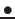CENTER FOR APPLIED MATHEMATICS AND THEORETICAL PHYSICS
UNIVERZA V MARIBORU UNIVERSITY OF MARIBOR
Phone +(386) (2) 2355 350 and 2355 351Fax +(386) (2) 2355 360
Robnik@uni-mb.siwww.camtp.uni-mb.si
PROF.DR. MARKO ROBNIK, DIRECTOR

Seminarsko predavanje
Centra za uporabno matematiko in teoretično fiziko

On the Dependence of Solutions and Eigenvalues on Potentials, Weights and Measures

Professor Dr. Meirong Zhang
Department of Mathematical Sciences & Zhou Pei-Yuan Center for Applied Mathematics, Tsinghua University, Beijing 100084, China
E-mail: mzhang@math.tsinghua.edu.cn

Abstract:

Differential equations usually contain finitely or infinitely dimensional parameters. In this talk I will address how solutions and eigenvalues will depend on infinitely dimensional parameters like potentials, weights, densities or distributions (measures). By considering the second-order ordinary differential equations and their generalizations, I will show that solutions and eigenvalues will continuously depend on these parameters in a very strong way, i.e. even when the weak topologies are considered, solutions and eigenvalues are continuous in these infinitely dimensional parameters. As an application, I will explain how such a strong continuity can be used to solve some infinitely dimensional extremal problems on eigenvalues which will yield optimal estimation of eigenvalues.

Seminarsko predavanje bo v torek 22. avgusta 2017 ob 16:00 uri v seminarski sobi CAMTP, Mladinska 3, drugo nadstropje levo. Vljudno vabljeni vsi zainteresirani, tudi študentje.

Prof.Dr. Marko Robnik
-- Direktor CAMTP --